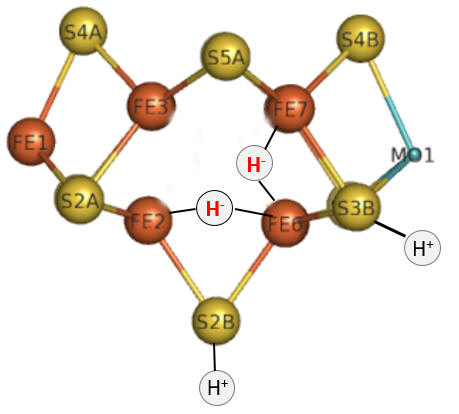# E3: Nitrogenase Reaction I - Addition of Electrons and Protons

$$\newcommand{\vecs}{\overset { \rightharpoonup} {\mathbf{#1}} }$$ $$\newcommand{\vecd}{\overset{-\!-\!\rightharpoonup}{\vphantom{a}\smash {#1}}}$$$$\newcommand{\id}{\mathrm{id}}$$ $$\newcommand{\Span}{\mathrm{span}}$$ $$\newcommand{\kernel}{\mathrm{null}\,}$$ $$\newcommand{\range}{\mathrm{range}\,}$$ $$\newcommand{\RealPart}{\mathrm{Re}}$$ $$\newcommand{\ImaginaryPart}{\mathrm{Im}}$$ $$\newcommand{\Argument}{\mathrm{Arg}}$$ $$\newcommand{\norm}{\| #1 \|}$$ $$\newcommand{\inner}{\langle #1, #2 \rangle}$$ $$\newcommand{\Span}{\mathrm{span}}$$ $$\newcommand{\id}{\mathrm{id}}$$ $$\newcommand{\Span}{\mathrm{span}}$$ $$\newcommand{\kernel}{\mathrm{null}\,}$$ $$\newcommand{\range}{\mathrm{range}\,}$$ $$\newcommand{\RealPart}{\mathrm{Re}}$$ $$\newcommand{\ImaginaryPart}{\mathrm{Im}}$$ $$\newcommand{\Argument}{\mathrm{Arg}}$$ $$\newcommand{\norm}{\| #1 \|}$$ $$\newcommand{\inner}{\langle #1, #2 \rangle}$$ $$\newcommand{\Span}{\mathrm{span}}$$

The sequential path of electrons from the reductase subunit containing the F cluster to the P and M clusters in the nitrogenase subunit should be apparent from the figures above. We will concentrate on the binding of N2 and how it receives electrons from the M cluster. The figure below shows the FeMo-cofactor and some adjacent amino acid residues. The Mo is not shown in space fill.Exercise $$\PageIndex{1}$$

If Val 70 is mutated to Ile, substrate appears not to access the cluster. Which part of the cluster most likely interacts with $$\ce{N2}$$?

TBA

Exercise $$\PageIndex{2}$$

His side chains are often found at enzyme active sites. What role might they play in catalysis?

TBA

The Lowe and Thorneley (LT) model has been proposed as a mechanism for dinitrogen reduction. In this model an electron and proton are added to the oxidized form of the enzyme (Eo) to produce E1. This is repeated 3 more times to form sequentially, E2, E3 and E4. Only then does N2 bind and the reduction of N2 occur. Two of the added electrons are accepted by H+ ions which form H2, which is liberated on N2 binding.Exercise $$\PageIndex{3}$$

Based on oxidation numbers of N and H in N2, NH3, H+ and H2 and the mechanism above, would you expect 8 electrons to be needed?

TBA

The crystal structure shows 2 ATPs bound to the reductase subunit. The stoichiometry of the reaction shows 16 ATP used. Simple math suggests that 2 ATP are cleaved to support the entry of one electron into the complex.

Part 1 - E1-E4: A potential structure for the E4 intermediate is shown below. Note the carbide is not shown. This is often called the Janus intermediate as it is half-way through the catalytic cycle. It is named for Janus, the Roman god of beginnings and transitions, and has been ascribed to gates, doors, doorways and passages. Janus is typically shown with two faces, one looking to the future and one to the past.The hydrides bridge 2 Fe ions so these are examples of three-center, two-electron bonds.

Exercise $$\PageIndex{1}$$

How can you tell that this figure represents E4?

Add answer text here and it will automatically be hidden if you have a "AutoNum" template active on the page.

How does this reaction occur? We must look to organometallic chemistry to help us understand the mechanism of this and subsequent steps. Clearly a hydride equivalent has been added to the metals, associated with the oxidation of metal ions in the center. This particular reaction is called an oxidative addition. Presumably the sulfur ions act as Lewis bases as they gain protons from a Lewis acid, probably HIs 195.

A figure showing oxidative additions for three different types of reactants are shown below. Note the specific example for insertion of H2, an example somewhat similar to the hydride additions to the M cluster. Oxidative reduction occurs most readily when the two oxidation states of the metal ion are stable. It is likewise favored for metal centers that are not sterically hindered (makes sense if A-B is to be added) and if A-B has a low bond dissociation energy.Exercise $$\PageIndex{5}$$

What role might the H+ ions have in the E4 complex shown above? Why might they be relatively close to the hydrides?

TBD

One way to study reaction intermediates is to trap them. If N2 can't access the binding site and the temperature is reduced, the accumulated hydrides and H+ in E4 might interact as the reaction goes back to E1.

Exercise $$\PageIndex{5}$$

What might be a likely product in such a case? What mutant might be used to attempt this experiment.